## The 1D Harmonic Oscillator

The harmonic oscillator is an extremely important physics problem. Many potentials look like a harmonic oscillator near their minimum. This is the first non-constant potential for which we will solve the Schrödinger Equation.

The harmonic oscillator Hamiltonian is given by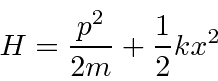which makes the Schrödinger Equation for energy eigenstates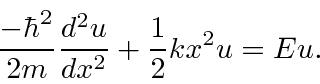Note that this potential also has a Parity symmetry. The potential is unphysical because it does not go to zero at infinity, however, it is often a very good approximation, and this potential can be solved exactly.

It is standard to remove the spring constant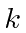from the Hamiltonian, replacing it with the classical oscillator frequency.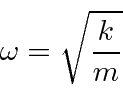The Harmonic Oscillator Hamiltonian becomes.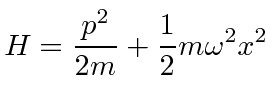The differential equation to be solved is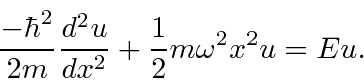To solve the Harmonic Oscillator equation, we will first change to dimensionless variables, then find the form of the solution for, then multiply that solution by a polynomial, derive a recursion relation between the coefficients of the polynomial, show that the polynomial series must terminate if the solutions are to be normalizable, derive the energy eigenvalues, then finally derive the functions that are solutions.

The energy eigenvalues are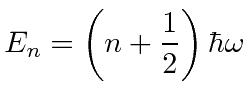for. There are a countably infinite number of solutions with equal energy spacing. We have been forced to have quantized energies by the requirement that the wave functions be normalizable.

The ground state wave function is.This is a Gaussian (minimum uncertainty) distribution. Since the HO potential has a parity symmetry, the solutions either have even or odd parity. The ground state is even parity.

The first excited state is an odd parity state, with a first order polynomial multiplying the same Gaussian.The second excited state is even parity, with a second order polynomial multiplying the same Gaussian.Note thatis equal to the number of zeros of the wavefunction. This is a common trend. With more zeros, a wavefunction has more curvature and hence more kinetic energy.

The general solution can be written aswith the coefficients determined by the recursion relationand the dimensionless variablegiven by.The series terminates with the last nonzero term having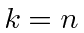.

Jim Branson 2013-04-22